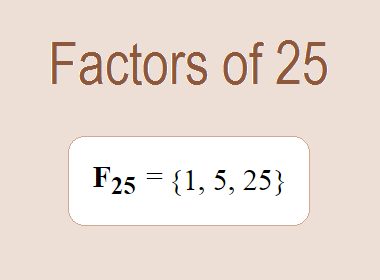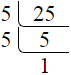# Factors of 25The factors of 25 are 1, 5, and 25 i.e. F25 = {1, 5, 25}. The factors of 25 are those numbers that can divide 25 without leaving a remainder.

We can check if these numbers are factors of 25 by dividing 25 by each of them. If the result is a whole number, then the number is a factor of 25. Let's do this for each of the numbers listed above:

·        1 is a factor of 25 because 25 divided by 1 is 25.

·        5 is a factor of 25 because 25 divided by 5 is 5.

·        25 is a factor of 25 because 25 divided by 25 is 1.

## How to Find Factors of 25?

1 and the number itself are the factors of every number. So, 1 and 25 are two factors of 25. To find the other factors of 25, we can start by dividing 25 by the numbers between 1 and 25. If we divide 25 by 2, we get a remainder of 1. Therefore, 2 is not a factor of 25. If we divide 25 by 3, we get a remainder of 1. Therefore, 3 is not a factor of 25.

Next, we can check if 4 is a factor of 25. If we divide 25 by 4, we get a remainder of 1. Therefore, 4 is not a factor of 25. If we divide 25 by 5, we get a remainder of 0. Therefore, 5 is a factor of 25. We can continue this process for all the possible factors of 25.

Through this process, we can find that the factors of 25 are 1, 5, and 25. These are the only numbers that can divide 25 without leaving a remainder.

********************

********************

## Properties of the Factors of 25

The factors of 25 have some interesting properties. One of the properties is that the sum of the factors of 25 is equal to 31. We can see this by adding all the factors of 25 together:

1 + 5 + 25 = 31

Another property of the factors of 25 is that they are all odd numbers. This is because 25 is an odd number, and any even number cannot divide an odd number without leaving a remainder.

Another property of the factors of 25 is that the only prime factor of 25 is 5.

## Applications of the Factors of 25

The factors of 25 have several applications in mathematics. One of the applications is in finding the highest common factor (HCF) of two or more numbers. The HCF is the largest factor that two or more numbers have in common. For example, to find the HCF of 25 and 35, we need to find the factors of both numbers and identify the largest factor they have in common. The factors of 25 are 1, 5, and 25. The factors of 35 are 1, 5, 7, and 35. The largest factor that they have in common is 5. Therefore, the HCF of 25 and 35 is 5.

Another application of the factors of 25 is in prime factorization. Prime factorization is the process of expressing a number as the product of its prime factors. The only prime factor of 25 is 5. We can express 25 as:

25 = 5 × 5

We can do prime factorization by division and factor tree method also. Here is the prime factorization of 25 by division method,25 = 5 × 5

Here is the prime factorization of 25 by the factor tree method,25 = 5 × 5## Conclusion

The factors of 25 are the numbers that can divide 25 without leaving a remainder. The factors of 25 are 1, 5, and 25. The factors of 25 have some interesting properties, such as being odd numbers and having a sum of 31. The factors of 25 have several applications in mathematics, such as finding the highest common factor and prime factorization.